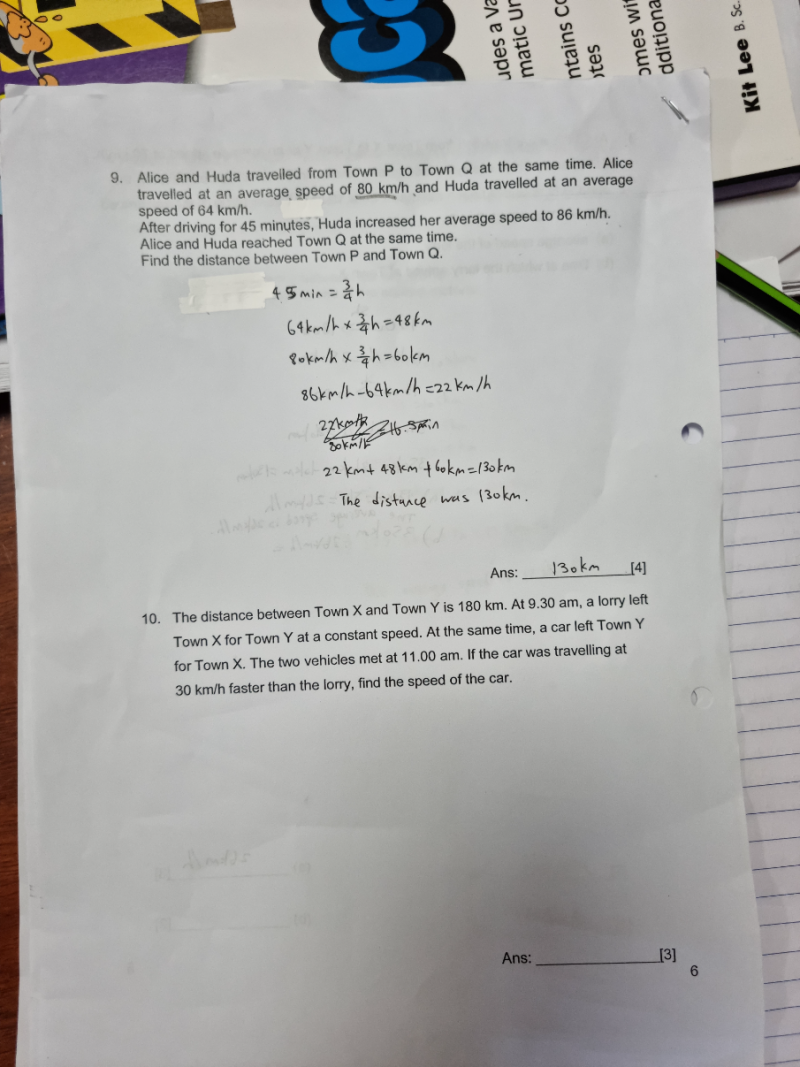# QuestionThank you.the top question did I get correct.thanks.the second question is a bit confused.thanks.

Question 10:

<——————180km———————–>

X |<—————–|——————————->| Y

Lorry(9:30)          11:00                                        Car(9:30)

Speed of car = u

Speed of lorry = u-30

11:00-9:30 =  (3/2)h        Using distance = speed x time,

(3/2)u + 3/2 (u-30) = 180  <——– x 2 on both sides

3u + 3u – 90 = 360

6u = 450  => u = 75

Hence speed of car is 75km/h

0 Replies 1 Like ✔Accepted Answer

Question 9:

Your working is partially correct. You start getting wrong when you add speed to distance.  They cannot be added together.

P  |——————————-|—————-|———————>|  Q

H<—-  12  –>A<——- u———>

A  = 80km/h   after  (3/4) h,   Distance travelled by A = 80 x  (3/4) =  60

H = 64km/h    after (3/4) h,    Distance travelled by H = 64 x (3/4) = 48

Distance between them after 45 mins = 60 – 48 = 12

Let u be the distance of A’s remaining journey to Q

Time taken by A to complete remaining journey = u/80

H increases speed to 86km/h for the remaining journey

Remaining journey of H = 12 + u

Time taken by H to complete remaining journey = (12+u)/86

They arrived at the same time at Q,

u/80 = (12+u)/86

86u = 80u + 960

6u = 960 => u = 160

Hence the distance between town P and Q = 60+160  or  48+12+160 = 220km